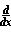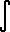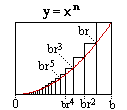Home    |    Teacher    |    Parents    |    Glossary    |    About UsEmail this page to a friendResources· Cool Tools · Formulas & Tables · References · Test Preparation · Study Tips · Wonders of MathSearchProof: Integral x^n/b> (Math | Calculus | Integrals | Table Of | x^n)

Discoverer: Cavalieri (1598-1647)

Proved: (n=1,2..9) Cavalieri; (n=positive integer) Fermat (1601-1665)Proof #1: From the derivative

Given :
1.x^m = m x^(m-1)
2. Fundamental Theorem of Calculusm x^(m-1) dx =../../derivatives/more/x^n.htmx^m dx = x^m + d. (Fundamental Theorem of Calculus) (d = an arbitrary constant)x^(m-1) dx = x^m / m + c (Divide both sides by m) (c=arbitrary constant, d/m = c)x^n dx = x^(n+1) / (n+1) + c (Set m=n+1, substitution) QED.Proof #2: Fermat's MethodKnown:
1. 1 + r + r^2 + .. + r^n = (1 - r^(n+1)) / (1-r)
2. 1 + r + r^2 + ... = 1 / (1-r) (r < 1)(0 to b) x^n dx is computed by taking the areas of an infinite number of unequal subintervals; larger subintervals at x close to b, smaller when close to 0.(0 to b) f(x) dx = f(b)*(b - br) + f(Br)*(Br - Br^2) + f(Br^2)*(Br^2 - Br^3) + ... (r -> 1-)
= b^n*(b - Br) + (Br)^n*(Br - Br^2) + (Br^2)^n*(Br^2 - Br^3) + ...
= b^(n+1)(1-r) + b^(n+1)r^(n+1)(1-r) + b^(n+1)r^(2n+2)(1-r) + ...
= b^(n+1)(1-r) [ 1 + r^(n+1) + (r^(n+1))^2 + ... ]
= b^(n+1)(1-r) [ 1 / (1-r^(n+1)) ] (Theorem 2.)
= b^(n+1) / [ (1 - r^(n+1)) / (1-r) ]
= b^(n+1) / [ 1 + r + r^2 + .. + r^n ] (Theorem 1.)
= b^(n+1) / (n+1) (r -> 1) QED.